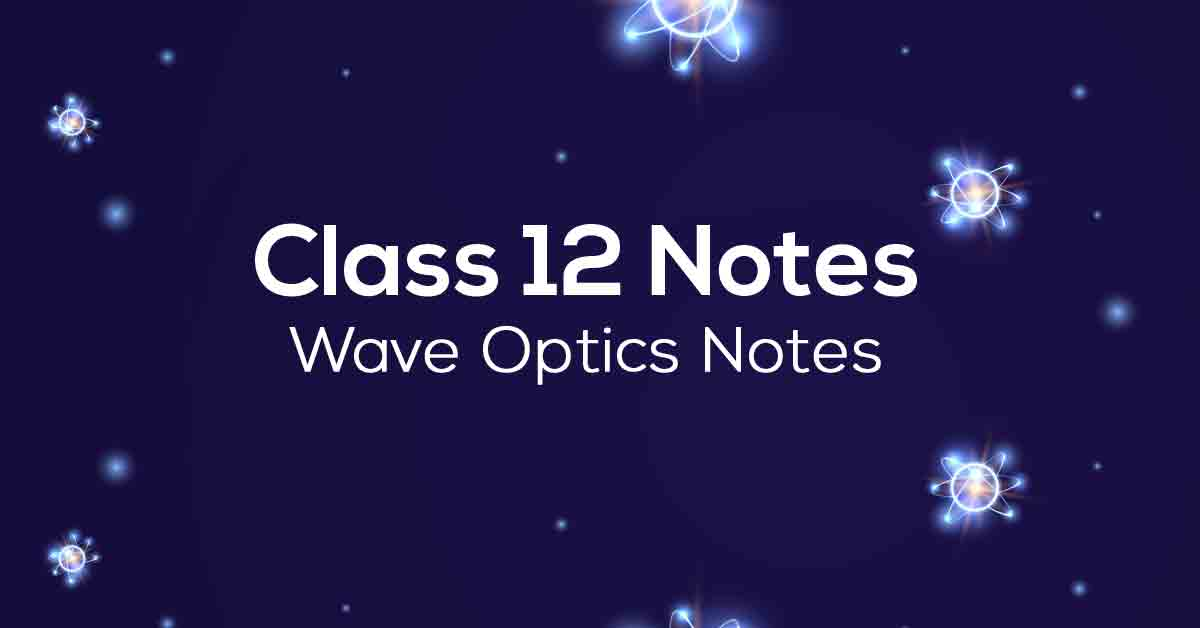Class 12th Physics Wave Optics NCERT Notes CBSE 2023

# Wave Optics Class 12 Notes# Chapter 10 Wave Optics

CBSE Grade 12 Wave Optics is one of the most significant and important chapters in Grade 12 Physics. In this chapter, students will learn about Huygen's principle, refraction, and reflection of waves using Huygen's code. , interference of light waves, etc. Students should practice the questions in the text with the help of NCERT notes to learn the nuances of writing answers and get the maximum score on the test.

Students can get free detailed notes for NCERT Physics Grade 12 Wave Optics from Vidyakul. In addition, in line with the latest CBSE testing and grading system, the Vidyakul notes provide comprehensive and up-to-date information from experienced instructors. Scroll down to learn more.

### Points to Remember

Some of the important points to remember from Class 12 Physics Chapter 10 are as follows:

• A wavefront is the locus of points in a propagating wave that oscillates in phase. As a result, a wavefront is defined as a surface with a constant phase.

• A point source’s wavefront will be spherical, while a distance source’s wavefront will be planar.

• The phenomenon of light refraction could not be explained by Newton’s corpuscular model of light, but it was successfully explained by Huygens’ wave nature of light.

• The Doppler effect refers to changes in light frequency caused by the motion of the source. But when the source moves toward the observer, the frequency rises (blue shift), and when the source moves away, the frequency falls (redshift).

• A plane-polarized wave is one in which all of the disturbances occur in the same plane.

• A polaroid is a material that allows disturbances only in one direction, known as the pass axis.

• The Brewster’s angle is the angle of incidence at which the reflected wave is completely polarised.

• The angular separation between two distant objects that can be clearly seen through a lens is given Δθ=0.61λ/a.

• Huygens’ wave theory can be used to verify the laws of reflection and refraction.

• Every point along a wave front acts as a light source, emitting secondary wavelets.

• Secondary wavelets spread with the speed of light in all directions in space (vacuum).

### Topics and Sub-topics

Students will learn various interesting topics like wavefronts, reflection and refraction of waves on a plane, and more in Chapter 10 of Grade 12 Physics. Students will also gain insight into Young's double slit experiment, edge width expressions, coherent sources, and diffraction for a single slit. However, students who have difficulty understanding the concepts of this chapter can refer to Vidyakul. Students should ensure that they fully understand the concepts of the chapter. Now, let's look at some important Wave Optics topics:

 Ex No Topic & Subtopics Under Wave Optics Description 10.1 Introduction 10.2 Huygens Principle 10.3 Refraction and Reflection of Plane Waves Using Huygens Principle 10.3.1 Refraction of a Plane Wave 10.3.2 Refraction at a Rarer Medium 10.3.3 Reflection of a Plane Wave by a Plane Surface 10.3.4 The Doppler Effect 10.4 Coherent and Incoherent Addition of Waves 10.5 Interference of Light Waves and Young’s Experiment 10.6 Diffraction 10.6.1 The Single Slit 10.6.2 Seeing the Single Slit Diffraction Pattern 10.6.3 Resolving Power Of Optical Instruments 10.6.4 The Validity Of Ray Optics 10.7 Polarisation 10.7.1 Polarisation By Scattering 10.7.2 Polarisation By Reflection

### Few Important Questions

• What is ‘Superposition’?

The superposition principle states that when two or more waves overlap in space, the resultant disturbance is equal to the algebraic sum of the individual disturbances.

• What is ‘Huygen’s Principle’?

Huygens's principle states that every point on a wavefront is in itself the source of spherical wavelets. The sum of these spherical wavelets forms the wavefront.

• What are the properties of waves?

There are many properties that scientists use to describe waves. They include amplitude, frequency, period, wavelength, speed, and phase.

### Practice Questions

In chapter 11 Physics in grade 12, students explore the dual nature of radiation and matter. Students can better understand the concepts by solving the exercises in the book using Chapter 11 of NCERT notes Physics 12. Students can follow the notes to understand the correct approach to answering the questions. Scroll down to read more about Chapter 11 Physics Grade 12 NCERT notes.